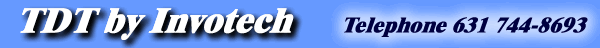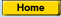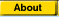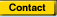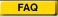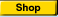TDT - Productivity solutions for tech illustrators.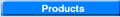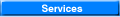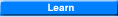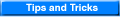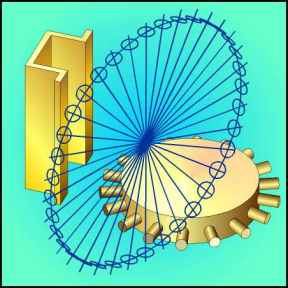Off Axis and Off Angle Projection - page 1 Certainly the single most asked "How do you …" question I have received since I started this series is about off angle and off axis projection. You know those annoying things that refuse to sit on one of the isometric planes. I’ll begin by dividing the exercise into three different problems with three different solutions. Case 1 is a projection that is off axis but on an isometric plane. Case 2 is a projection that is off axis and not on an isometric plane. Click here Case 3 is a projection that is off axis and on a tangent to the isometric plane. Click here Off axis on a tangent to a non isometric plane will not deal with in this series. While one solution to all three problems is possible, the first two cases can be resolved by much simpler means. A successful illustrator is be able to picture what an off angle object looks like before they begin to draw.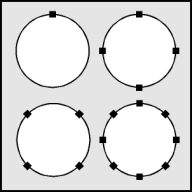Before we can begin you must create a set of master ellipses. Create a perfect circle by choosing the ellipse tool (F7) and while holding down the constrain key (ctrl) drag across the screen to about 3/8 inch (Watch the status line). Convert this to a curve (ctrl+Q) to place 4 nodes around the circle then rotate the circle 45 degrees to place the nodes along the isometric axis’s. Use the Node Edit Roll-up (ctrl+F10) and add (Plus sign) nodes. This places nodes at the major and minor axis’s.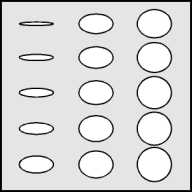Use the Transform Roll-up to apply to a duplicate the following: Note: Users of Tech Drawing Tools drag the appropriate ellipse from the Off Axis Symbol Library. Place a text note inside each ellipse denoting it’s degree. 5 degree ellipse = 10% vertical scale. 10 degree ellipse = 18% vertical scale. 15 degree ellipse = 26% vertical scale. 20 degree ellipse = 34% vertical scale. 30 degree ellipse = 50% vertical scale. Isometric ellipse (35 degree 16 minutes) = 57.4% vertical scale. 40 degree ellipse = 63% vertical scale. 45 degree ellipse = 70% vertical scale. 50 degree ellipse = 76% vertical scale. 55 degree ellipse = 79% vertical scale. 60 degree ellipse = 83% vertical scale. 65 degree ellipse = 88% vertical scale. 70 degree ellipse = 94% vertical scale. 80 degree ellipse = 97% vertical scale. 90 degree ellipse = the perfect circle. Other ellipses can be created by extrapolating the above table. Move all of these ellipses off to the side.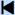Start 1 2 3 4 5 6 7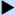Next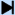End Learn More